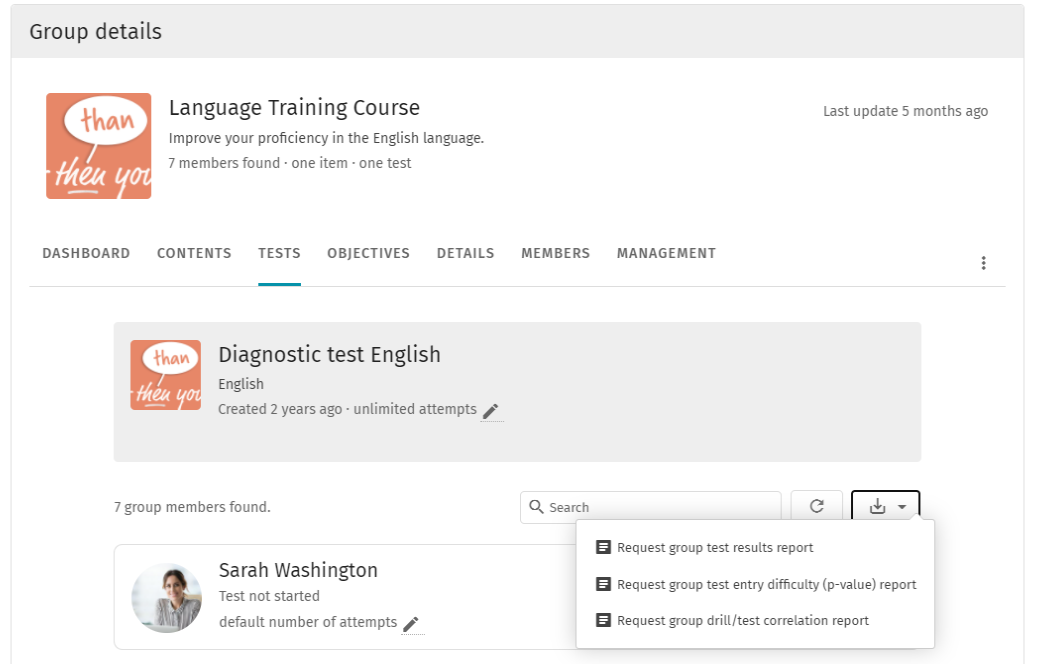# P-value report

How difficult or how easy were the questions within a test? The p-value of a test question provides insight into this. For each test you can download a p-value report and analyze the difficulty of your questions, dilemmas or case studies.

The p-value of a question can be calculated with the following formula:

p-value = number of participants that answered the question correctly / the total number of participants that answered the question
Low p-value
A low p-value means that few participants answer the question correctly. This can mean that a question is too difficult for the participants, but it can also mean that there is a mistake in the question itself. Either of these would be enough reason to take another close look at the question.
High p-value
A high p-value means that a lot of participants correctly answered the question. This may be because the question is formulated too easily, where, for example, you've written bad distractions. Do you want to know How to write good distractions when creating content in Drillster? Then click here

A p-value between 0.3 and 0.8 is optimal. Searching for outliers inside a test can provide valuable information. If we assume that a test contains questions on the same subject, then there should be no major outliers on the high or low end of the spectrum, because you assume that the participant scores similarly on different questions of one subject.

For tests, the p-value can be calculated over the answers of all participants within a group, but also over all the answers of all participants within all groups that have ever made the same test.

Below you can see where to get a p-value report.

• Click on the button 'view results' at the desired test.
• Then click on the button as shown in the image below.
• To retrieve the p-value report, choose the option 'Request group test entry difficulty (p-value) report'.